Updating search results...

# 16 Results

View
Selected filters:
• Shodor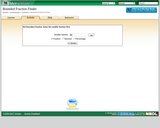Unrestricted Use
CC BY
Rating
0.0 stars

Determine the value of two fractions you have chosen (which are represented as points on a number line). Then find a fraction whose value is between your two fractions and determine its value. This manipulative is the same as "Bounded Fraction Pointer" but there is no arrow to help the user determine the value of a fraction between the two endpoints. Bounded Fraction Finder is one of the Interactivate assessment explorers.

Subject:
Mathematics
Material Type:
Activity/Lab
Diagram/Illustration
Formative Assessment
Interactive
Lesson Plan
Self Assessment
Simulation
Provider:
Shodor
Author:
Lindsay Reiten
02/14/2017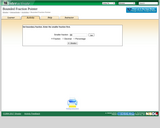Restricted Use
Rating
0.0 stars

Determine the value of two fractions you have chosen (which are represented as points on a number line). Then find a fraction whose value is between your two fractions (using an arrow on the number line as a guide) and determine its value. Bounded Fraction Pointer is one of the Interactivate assessment explorers.

Subject:
Mathematics
Material Type:
Activity/Lab
Diagram/Illustration
Formative Assessment
Interactive
Lesson Plan
Self Assessment
Simulation
Provider:
Shodor
02/14/2017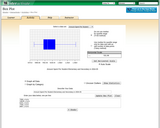Restricted Use
Rating
0.0 stars

This activity allows the user to view box plots for either built-in or user-specified data, and experiment with outliers.

Subject:
Mathematics
Material Type:
Activity/Lab
Diagram/Illustration
Formative Assessment
Interactive
Lesson Plan
Simulation
Provider:
Shodor
11/30/2016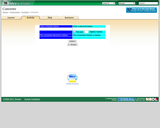Restricted Use
Rating
0.0 stars

Converts fractions to decimals and decimals to fractions. Observe the relationships between fractions and decimals.

Subject:
Mathematics
Material Type:
Activity/Lab
Interactive
Lesson Plan
Self Assessment
Simulation
Provider:
Shodor
02/14/2017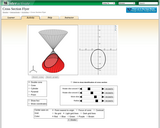Restricted Use
Rating
0.0 stars

This activity allows the user to explore different cross sections of various cones, cylinders, prisms, and pyramids. Cross sections can be difficult for students to understand, given that the concept requires that students switch between three-dimensional shapes and two-dimensional representations of those shapes.

Subject:
Mathematics
Material Type:
Activity/Lab
Diagram/Illustration
Formative Assessment
Interactive
Lesson Plan
Self Assessment
Simulation
Provider:
Shodor
11/03/2016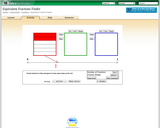Restricted Use
Rating
0.0 stars

Visually represent two unique fractions that are equivalent to a given fraction. The fractional value is shown on a number line after you check to see if your fraction is correct. Equivalent Fraction Finder is one of the Interactivate assessment explorers.

Subject:
Mathematics
Material Type:
Activity/Lab
Diagram/Illustration
Formative Assessment
Interactive
Lesson Plan
Self Assessment
Simulation
Provider:
Shodor
02/14/2017Restricted Use
Rating
0.0 stars

Visually represent two unique fractions that are equivalent to a given fraction. The fractional value is shown on a number line as you color in the fraction. Equivalent Fraction Pointer is one of the Interactivate assessment explorers.

Subject:
Mathematics
Material Type:
Activity/Lab
Diagram/Illustration
Formative Assessment
Interactive
Lesson Plan
Self Assessment
Simulation
Provider:
Shodor
02/14/2017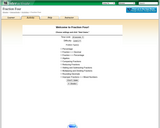Restricted Use
Rating
0.0 stars

This applet allows the students to learn to multiply, add, simplify and convert fractions, decimals and percents by playing a game.

Subject:
Mathematics
Material Type:
Activity/Lab
Diagram/Illustration
Formative Assessment
Interactive
Lesson Plan
Self Assessment
Simulation
Provider:
Shodor
02/14/2017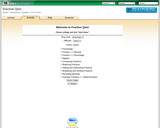Restricted Use
Rating
0.0 stars

This applet allows the students to learn to multiply, add, simplify, and convert fractions, decimals, and percents by playing a game.

Subject:
Mathematics
Material Type:
Activity/Lab
Diagram/Illustration
Formative Assessment
Interactive
Lesson Plan
Self Assessment
Provider:
Shodor
02/14/2017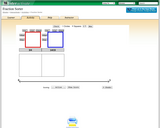Restricted Use
Rating
0.0 stars

This activity allows the user to practice fractions and get a better understanding of them. They get a chance to work with two different aspects of fractions: making them and putting them in order from least to greatest.

Subject:
Mathematics
Material Type:
Activity/Lab
Diagram/Illustration
Formative Assessment
Interactive
Lesson Plan
Self Assessment
Simulation
Provider:
Shodor
02/14/2017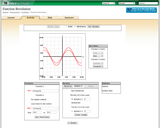Restricted Use
Rating
0.0 stars

This applet is an expanded version of 3D Transmographer. This 3-dimensional model allows users to explore functions and their revolution around an axis. Once one or two functions have been rotated around an axis, the applet calculates the surface area and volume for the resulting solid. By clicking and dragging on the graph, users can change the vantage point from which they view the graph in order to better understand the functions, the revolution, and the resulting solid.

Subject:
Mathematics
Material Type:
Activity/Lab
Diagram/Illustration
Formative Assessment
Interactive
Lesson Plan
Self Assessment
Simulation
Provider:
Shodor
11/03/2016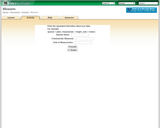Restricted Use
Rating
0.0 stars

This applet allows the user to select the number and height of plants or to name a different item data have been collected about to experiment with to find the total height, mean, median, mode, range, variance, and deviation.

Subject:
Mathematics
Material Type:
Activity/Lab
Diagram/Illustration
Formative Assessment
Interactive
Simulation
Provider:
Shodor
11/30/2016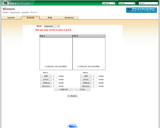Restricted Use
Rating
0.0 stars

Mixtures allows the user to explore percent problems with two sets of circles. Each set of circles can have both colored and / or uncolored circles. The number of colored circles represents the percent of each pile. The user can select from a four modes: Exploration, Unknown Pile, Unknown Percent, or Unknown total.

Subject:
Mathematics
Material Type:
Activity/Lab
Diagram/Illustration
Formative Assessment
Interactive
Lesson Plan
Self Assessment
Simulation
Provider:
Shodor
11/30/2016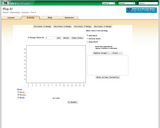Restricted Use
Rating
0.0 stars

PlopIt allows users to build dot plots of data using the mouse. View how the mean, median, and mode change as entries are added to the plot. Parameters: Range for observations.

Subject:
Mathematics
Material Type:
Activity/Lab
Diagram/Illustration
Formative Assessment
Interactive
Lesson Plan
Simulation
Provider:
Shodor
11/30/2016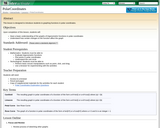Rating
0.0 stars

This lesson is designed to introduce students to graphing functions in polar coordinates.
Upon completion of this lesson, students will:
Have a basic understanding of the graphs of trigonometric functions in polar coordinatesUnderstand how certain changes to the function affect the graph

Subject:
Mathematics
Material Type:
Diagram/Illustration
Simulation
Provider:
The Shodor Education Foundation
03/20/2018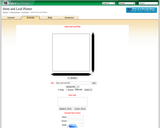Restricted Use
Rating
0.0 stars

This activity displays a stem and leaf plot representing data either entered by the user or generated by the computer. The user can use the applet as a tool that creates a stem and leaf plot from data the user inputs, or to practice calculating the mean, median, and mode of a given data set.

Subject:
Mathematics
Material Type:
Activity/Lab
Diagram/Illustration
Formative Assessment
Interactive